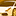cancel
Showing results for
Did you mean:

## Logging when using multiprocessing with joblibNew Contributor II

Hi,

I'm using joblib for multiprocessing in one of our processes. The logging does work well (except weird py4j errors which I supress) except when it's within multiprocessing. Also how do I supress the other errors that I always receive on DB - perhaps is there some guide on this? Thanks

`````` 2023-04-17 11:12:32 [INFO] - Starting multiprocessing
2023-04-17 11:12:33 [INFO] - Exception while sending command.
Traceback (most recent call last):
File "/databricks/spark/python/lib/py4j-0.10.9.5-src.zip/py4j/clientserver.py", line 503, in send_command
self.socket.sendall(command.encode("utf-8"))
ConnectionResetError: [Errno 104] Connection reset by peer

During handling of the above exception, another exception occurred:

Traceback (most recent call last):
File "/databricks/spark/python/lib/py4j-0.10.9.5-src.zip/py4j/java_gateway.py", line 1038, in send_command
response = connection.send_command(command)
File "/databricks/spark/python/lib/py4j-0.10.9.5-src.zip/py4j/clientserver.py", line 506, in send_command
raise Py4JNetworkError(
py4j.protocol.Py4JNetworkError: Error while sending
Squares: [1, 4, 9, 16, 25]``````
``````import logging
import os
from joblib import Parallel, delayed
from time import sleep

def setup_logging():
logging.basicConfig(level=logging.INFO,
format="%(asctime)s [%(levelname)s] - %(message)s",
datefmt="%Y-%m-%d %H:%M:%S")
logging.getLogger().setLevel(logging.INFO)
pyspark_log = logging.getLogger('pyspark')
pyspark_log.setLevel(logging.WARNING)
logging.getLogger("py4j").setLevel(logging.WARNING)

def calculate_square(number):
sleep(1)  # Simulate a time-consuming task
result = number ** 2
logging.info(f"Square of {number} is {result}")
return result

setup_logging()
logging.info(f"Starting multiprocessing")
# List of numbers to calculate squares
numbers = [1, 2, 3, 4, 5]

# Using joblib for multiprocessing
n_jobs = os.cpu_count()
results = Parallel(n_jobs=n_jobs)(delayed(calculate_square)(num) for num in numbers)

print(f"Squares: {results}")``````Anonymous
Not applicable

@Sam G​ :

It seems like the issue is related to the py4j library used by Spark, and not specifically related to joblib or multiprocessing. The error message indicates a network error while sending a command between the Python process and the Java Virtual Machine (JVM) running Spark.

To suppress the error messages, you can add the following lines of code to your setup_logging function:

``````logging.getLogger("py4j").setLevel(logging.ERROR)
logging.getLogger("py4j.java_gateway").setLevel(logging.ERROR)``````

This will set the log level of the py4j and py4j.java_gateway modules to ERROR, which will suppress their log messages.

Regarding the issue with logging within multiprocessing, you can try using a QueueHandler and QueueListener to send log messages from child processes back to the parent process, where they can be logged normally. Here's an example:

``````import logging
import os
from joblib import Parallel, delayed
from multiprocessing import Queue, current_process
from logging.handlers import QueueHandler, QueueListener
from time import sleep

def setup_logging():
logging.basicConfig(level=logging.INFO,
format="%(asctime)s [%(levelname)s] - %(message)s",
datefmt="%Y-%m-%d %H:%M:%S")
logging.getLogger().setLevel(logging.INFO)
pyspark_log = logging.getLogger('pyspark')
pyspark_log.setLevel(logging.WARNING)
logging.getLogger("py4j").setLevel(logging.ERROR)
logging.getLogger("py4j.java_gateway").setLevel(logging.ERROR)

# Create a queue handler and listener for logging in child processes
log_queue = Queue(-1)
queue_handler = QueueHandler(log_queue)
queue_listener = QueueListener(log_queue, logging.getLogger())

# Start the queue listener in a separate thread
queue_listener.start()

def calculate_square(number, log_queue):
sleep(1)  # Simulate a time-consuming task
result = number ** 2
logging.info(f"[{current_process().name}] Square of {number} is {result}")
log_queue.put(f"[{current_process().name}] Square of {number} is {result}")
return result

setup_logging()
logging.info(f"Starting multiprocessing")
# List of numbers to calculate squares
numbers = [1, 2, 3, 4, 5]

# Using joblib for multiprocessing
n_jobs = os.cpu_count()

# Create a queue for logging in child processes
log_queue = Queue(-1)

# Create a delayed function with the log_queue as an argument
def delayed_func(num):
return delayed(calculate_square)(num, log_queue)

results = Parallel(n_jobs=n_jobs)(delayed_func(num) for num in numbers)

# Stop the queue listener once all child processes have finished logging
queue_listener.stop()

print(f"Squares: {results}")``````

This code creates a queue handler and listener for logging in child processes, and passes the queue to the calculate_square function as an argument. The function logs the square calculation to both the local logger and the queue, which is then processed by the listener running in the main process.

Note that the queue listener should be stopped once all child processes have finished logging, which is done in the example code using the queue_listener.stop() method.Welcome to Databricks Community: Lets learn, network and celebrate together

Join our fast-growing data practitioner and expert community of 80K+ members, ready to discover, help and collaborate together while making meaningful connections.